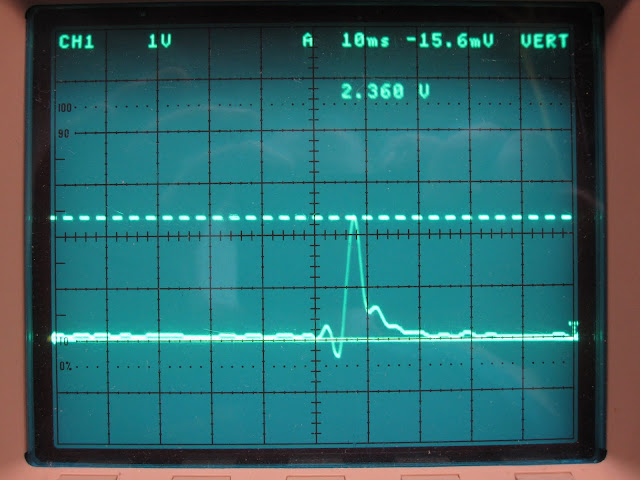## Discrepancy Between Circuit Simulation and Reality

29 09 2011

Previously we modeled the polywell coils and power supply in SPICE.

Today I returned to that model.

All resistance values in the simulation are based on real world measurements with the exception of coil inductance (code).

Starting with an estimate for coil inductance of 0.1 mH the discharge current looks like this:The simulation’s peak of 1.5 kA is nowhere near the 2.3 kA we are getting in the real world:OK. Maybe the value for coil inductance is off?

I played around with the value for coil inductance but the simulation would not match reality.

As a control I replaced the simulated inductor with a 1 mΩ resistor (code). Looks like this:The simulation predicts ~1.8 kA but in reality we see 2.3kA!

Where does this discrepancy come from?

UPDATE: Reader Andrew solved the mystery:

You could try changing the ON resistance of your switch/SCR to something a bit lower than 100mOhms

.model MySwitch SW(Ron=.1 Roff=1Meg Vt=3 Vh=0)

I can’t see the part number of your SCR but 2mOhms would seem reasonable.

I didn’t notice that rather high resistance lurking in the SCR model.

Now the simulation matches reality very closely with 0.06mH coil inductance (code):Good work Andrew and the rest of the internet brain!

Advertisements

### 5 responses

29 09 2011

I think it is most likely due to the stray capacitance throughout the circuit. The coil will also have interwinding capacitance which will make it look somewhat like a resonant tank circuit – so this resonance probably is where the extra complicated real world behavior that you’re seeing is coming from.

The trick is to treat any real device as a blend of series resistance, series inductance and parallel capacitance.

eg:
A real capacitor is mostly capacitance, but in practice you need to be aware of it’s internal equivalent series resistance, because this models the dissipation the non-ideal component has, and it limits the power the cap can handle for long.
A real coil is mostly inductance, but it also has that interwinding capacitance, which will make it look resonant at some frequency.
A real resistor even has some inductance.
All of these always happen – empty space has some minimal capacitance per length and inductance per length – this is how it can carry light.
You mostly don’t need to worry about this except at really high frequencies – at low frequencies (wire length < ~ 5 wavelengths, where speed in a wire usually ~ 2/3c ) you can use the "lumped" parameter model, IE – what SPICE does.

So, you should be able to measure the impedance of your coil, using a frequency generator and an oscilloscope. (impedance, btw = complex resistance, ie, Z (impedance, ohms) = R(resistance, real, ohms) + j (j^2=-1) times X (reactance, ohms).

So impedance for an ideal coil is Z_L = j*omega*L, For a cap, Z_C = -j /(omega*C), Where omega = 2*pi*(frequency in Hz), L=inductance in Henries, C = capacitance in Farads. Doing this lets you pretend that ideal caps and ideal coils are just resistors, albeit ones with purely imaginary resistance. (this is why they don't dissipate power – they just cause the relationship between Voltage and Current to change phase, but ohms law V = IR still holds, except now v(t)=i(t)*Z().)

I suspect that when your Polywell captures electrons, it's impedance may change… possibly even in a complicated manner. I don't have any idea, at the moment, what that may look like, and I've even less of an idea what the impedance behavior of your electron injection circuit will look like in operation. This right here is why your project is so interesting – even if you don't reach break-even.

In a functioning Polywell power reactor, these things will likely need to be known – to be able to control the depth of the well, whilst maintaining that critical beta=1 condition.

29 09 2011

Oh, also – this resonance stuff can also affect your CRO leads, so that what the current is really doing isn’t what it seems to be doing… Just to add to the confusion. All of the above is really the essence of the black art of radio-frequency design. You can hopefully avoid the worst by sticking to lumped-parameter models. (and not have to consider distributed-parameter models like the transmission line components in spice)

29 09 2011

You might want to try modeling the capacitance of the sensing circuit. As others have said, the stray capacitance could cause the voltage you are picking up to be higher than the actual current through the thing.

29 09 2011

You could try changing the ON resistance of your switch/SCR to something a bit lower than 100mOhms

.model MySwitch SW(Ron=.1 Roff=1Meg Vt=3 Vh=0)

I can’t see the part number of your SCR but 2mOhms would seem reasonable.

30 09 2011

THAT’S IT!